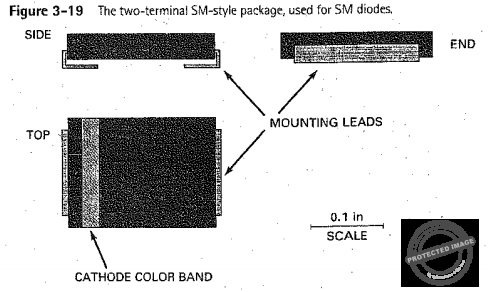# Malvino: MCQ in Diode Theory

(Last Updated On: September 29, 2020)

This is the Multiple Choice Questions in Chapter 3: Diode Theory from the book Electronic Principles 7th Edition by Albert Malvino. If you are looking for a reviewer in Electronics Engineering this will definitely help. I can assure you that this will be a great help in reviewing the book in preparation for your Board Exam. Make sure to familiarize each and every questions to increase the chance of passing the ECE Board Exam.

### Topic Outline

• MCQs in Basic Ideas
• MCQs in the Ideal Diode
• MCQs in the Second Approximation
• MCQs in the Third Approximation
• MCQs in Up-Down Circuit Analysis
• MCQs in How to calculate Bulk Resistance
• MCQs in DC Resistance of a Diode
• MCQs in Surface-Mount Diodes

### Start Practice Exam Test Questions

Choose the letter of the best answer in each questions.

1 . When the graph of current versus voltage is a straight line, the device is referred to as

• a. Active
• b. Linear
• c. Nonlinear
• d. Passive

2. What kind of device is a resistor?

• a. Unilateral
• b. Linear
• c. Nonlinear
• d. Bipolar

3. What kind of a device is a diode?

• a. Bilateral
• b. Linear
• c. Nonlinear
• d. Unipolar

4. How is a non-conducting diode biased?

• a. Forward
• b. Inverse
• c. Poorly
• d. Reverse

5. When the diode current is large, the bias is

• a. Forward
• b. Inverse
• c. Poor
• d. Reverse

6. The knee voltage of a diode is approximately equal to the

• a. Applied voltage
• b. Barrier potential
• c. Breakdown voltage
• d. Forward voltage

7. The reverse current consists of minority-carrier current and

• a. Avalanche current
• b. Forward current
• c. Surface-leakage current
• d. Zener current

8. How much voltage is there across the second approximation of a silicon diode when it is forward biased?

• a. 0
• b. 0.3 V
• c. 0.7 V
• d. 1 V

9. How much current is there through the second approximation of a silicon diode when it is reverse biased?

• a. 0
• b. 1 mA
• c. 300 mA
• d. None of the above

10. How much forward diode voltage is there with the ideal diode approximation?

• a. 0
• b. 0.7 V
• c. More than 0.7 V
• d. 1 V

11. The bulk resistance of a 1N4001 is

• a. 0
• b. 0.23 ohm
• c. 10 ohm
• d. 1 kohm

12. If the bulk resistance is zero, the graph above the knee becomes

• a. Horizontal
• b. Vertical
• c. Tilted at 450
• d. None of the above

13. The ideal diode is usually adequate when

• a. Troubleshooting
• b. Doing precise calculations
• c. The source voltage is low
• d. The load resistance is low

14. The second approximation works well when

• a. Troubleshooting
• b. Load resistance is high
• c. Source voltage is high
• d. All of the above

15. The only time you have to use the third approximation is when

• a. Load resistance is low
• b. Source voltage is high
• c. Troubleshooting
• d. None of the above

16. How much load current is there in Fig. 3-19 (see your textbook) with the ideal diode?

• a. 0
• b. 14.3 mA
• c. 15 mA
• d. 50 mA

17. How much load current is there in Fig. 3-19 (see your textbook) with the second approximation?

• a. 0
• b. 14.3 mA
• c. 15 mA
• d. 50 mA

18. How much load current is there in Fig. 3-19 with the third approximation?

• a. 0
• b. 14.3 mA
• c. 15 mA
• d. 50 mA

19. If the diode is open in Fig. 3-19, the load voltage is

• a. 0
• b. 14.3 V
• c. 20 V
• d. -15 V

20. If the resistor is ungrounded in Fig. 3-19, the voltage measured with a DMM between the top of the resistor and ground is closest to

• a. 0
• b. 15 V
• c. 20 V
• d. -15 V

21. The load voltage measures zero in Fig. 3-19. The trouble may be

• a. A shorted diode
• b. An open diode
• c. An open load resistor
• d. Too much supply voltage### Complete List of MCQ in Electronic Principles by Albert Malvino

P inoyBIX educates thousands of reviewers and students a day in preparation for their board examinations. Also provides professionals with materials for their lectures and practice exams. Help me go forward with the same spirit.

“Will you subscribe today via YOUTUBE?”

Subscribe

• Full Content Access Exclusive to Premium members

## PINOYBIX FREEBIES FOR PREMIUM MEMBERSHIP:

• CIVIL ENGINEERING REVIEWER
• CIVIL SERVICE EXAM REVIEWER
• CRIMINOLOGY REVIEWER
• ELECTRONICS ENGINEERING REVIEWER (ECE/ECT)
• ELECTRICAL ENGINEERING & RME REVIEWER
• FIRE OFFICER EXAMINATION REVIEWER
• LET REVIEWER
• MASTER PLUMBER REVIEWER
• MECHANICAL ENGINEERING REVIEWER
• NAPOLCOM REVIEWER

## FOR A LIMITED TIME

If you subscribe for PREMIUM today!

PinoyBIX Engineering. © 2014-2023 All Rights Reserved | How to Donate? |